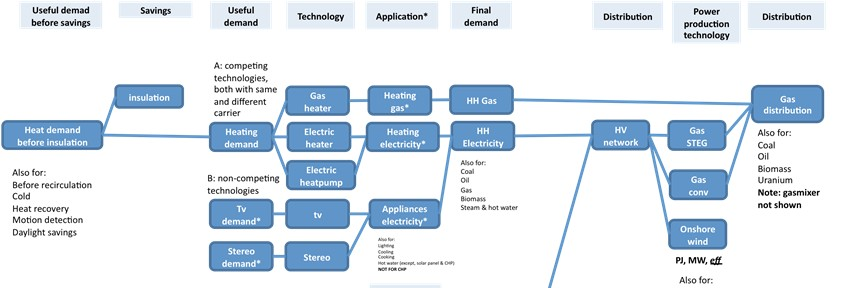# Energy calculations

Energy is the 'common currency' of the ETM. When you change a slider, you effectively change the energy flow in the ETM. This energy flow can be represented as a graph such as shown below:The nodes are represented by nodes which convert or transport energy (possibly with loss). The edges (connections) are the energy flows which are characterized by volume (in megajoule) and carrier type (such as coal, electricity, useable_heat etc.).

The ETM is demand driven meaning that moving a slider in the demand sector, the graph is traverse from left to right. From 'useful demand' (heating, hot water, car kilometers) to 'primary demand' (the extraction of gas, import of coal etc.).